# US9928214B2 - Sketching structured matrices in nonlinear regression problems - Google Patents

## Info

Publication number
US9928214B2
US9928214B2 US14/333,978 US201414333978A US9928214B2 US 9928214 B2 US9928214 B2 US 9928214B2 US 201414333978 A US201414333978 A US 201414333978A US 9928214 B2 US9928214 B2 US 9928214B2
Authority
US
United States
Prior art keywords
matrix
ε
vector
nonlinear regression
image
Prior art date
Legal status (The legal status is an assumption and is not a legal conclusion. Google has not performed a legal analysis and makes no representation as to the accuracy of the status listed.)
Active, expires
Application number
US14/333,978
Other versions
US20150317282A1 (en
Inventor
Haim Avron
Vikas Sindhwani
David P. Woodruff
Current Assignee (The listed assignees may be inaccurate. Google has not performed a legal analysis and makes no representation or warranty as to the accuracy of the list.)
Original Assignee
Priority date (The priority date is an assumption and is not a legal conclusion. Google has not performed a legal analysis and makes no representation as to the accuracy of the date listed.)
Filing date
Publication date
Priority to US201361911786P priority Critical
Application filed by International Business Machines Corp filed Critical International Business Machines Corp
Assigned to INTERNATIONAL BUSINESS MACHINES CORPORATION reassignment INTERNATIONAL BUSINESS MACHINES CORPORATION ASSIGNMENT OF ASSIGNORS INTEREST (SEE DOCUMENT FOR DETAILS). Assignors: AVRON, HAIM, SINDHWANI, VIKAS, WOODRUFF, DAVID P.
Priority to US14/333,978 priority patent/US9928214B2/en
Assigned to AFRL/RIJ reassignment AFRL/RIJ CONFIRMATORY LICENSE (SEE DOCUMENT FOR DETAILS). Assignors: INTERNATIONAL BUSINESS MACHINE CORPORATION
Publication of US20150317282A1 publication Critical patent/US20150317282A1/en
Publication of US9928214B2 publication Critical patent/US9928214B2/en
Application granted granted Critical
Application status is Active legal-status Critical

• 239000011159 matrix materials Substances 0 abstract claims description 141
• 238000004590 computer program Methods 0 abstract claims description 13
• 238000003860 storage Methods 0 claims description 21
• 239000000047 products Substances 0 claims description 14
• 239000002609 media Substances 0 claims description 13
• 230000015654 memory Effects 0 claims description 12
• 230000000875 corresponding Effects 0 claims description 10
• 230000000051 modifying Effects 0 claims description 3
• 238000009740 moulding (composite fabrication) Methods 0 claims 2
• 238000004422 calculation algorithm Methods 0 description 25
• 230000000996 additive Effects 0 description 15
• 239000000654 additives Substances 0 description 15
• 230000003993 interaction Effects 0 description 6
• 238000009826 distribution Methods 0 description 5
• 238000000034 methods Methods 0 description 5
• 238000005070 sampling Methods 0 description 5
• 230000001721 combination Effects 0 description 4
• 238000004891 communication Methods 0 description 4
• 239000011162 core materials Substances 0 description 4
• 230000002829 reduced Effects 0 description 4
• 238000003672 processing method Methods 0 description 3
• 230000001603 reducing Effects 0 description 3
• 230000018109 developmental process Effects 0 description 2
• 238000007429 general methods Methods 0 description 2
• 230000001965 increased Effects 0 description 2
• 230000003287 optical Effects 0 description 2
• 230000001902 propagating Effects 0 description 2
• 238000006722 reduction reaction Methods 0 description 2
• 230000001133 acceleration Effects 0 description 1
• 238000010276 construction Methods 0 description 1
• 239000010949 copper Substances 0 description 1
• 230000001186 cumulative Effects 0 description 1
• 238000000354 decomposition Methods 0 description 1
• 230000001419 dependent Effects 0 description 1
• 238000005315 distribution function Methods 0 description 1
• 239000000835 fiber Substances 0 description 1
• 238000007667 floating Methods 0 description 1
• 238000009499 grossing Methods 0 description 1
• 230000001976 improved Effects 0 description 1
• 239000011133 lead Substances 0 description 1
• 230000000670 limiting Effects 0 description 1
• 239000011572 manganese Substances 0 description 1
• 238000004519 manufacturing process Methods 0 description 1
• 230000004048 modification Effects 0 description 1
• 238000006011 modification Methods 0 description 1
• 230000002093 peripheral Effects 0 description 1
• 239000004065 semiconductor Substances 0 description 1
• 230000003068 static Effects 0 description 1
• 238000000844 transformation Methods 0 description 1
• 230000001131 transforming Effects 0 description 1

## Images

•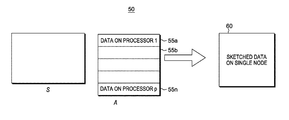•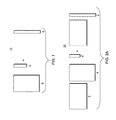•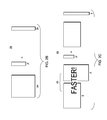•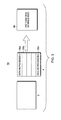•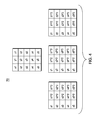•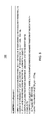•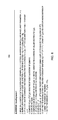•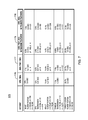•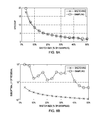•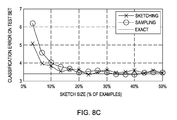•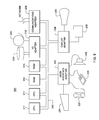## Classifications

• GPHYSICS
• G06COMPUTING; CALCULATING; COUNTING
• G06FELECTRIC DIGITAL DATA PROCESSING
• G06F17/00Digital computing or data processing equipment or methods, specially adapted for specific functions
• G06F17/10Complex mathematical operations
• G06F17/18Complex mathematical operations for evaluating statistical data, e.g. average values, frequency distributions, probability functions, regression analysis
• GPHYSICS
• G06COMPUTING; CALCULATING; COUNTING
• G06FELECTRIC DIGITAL DATA PROCESSING
• G06F17/00Digital computing or data processing equipment or methods, specially adapted for specific functions
• G06F17/10Complex mathematical operations
• GPHYSICS
• G06COMPUTING; CALCULATING; COUNTING
• G06FELECTRIC DIGITAL DATA PROCESSING
• G06F17/00Digital computing or data processing equipment or methods, specially adapted for specific functions
• G06F17/10Complex mathematical operations
• G06F17/16Matrix or vector computation, e.g. matrix-matrix or matrix-vector multiplication, matrix factorization
• GPHYSICS
• G06COMPUTING; CALCULATING; COUNTING
• G06FELECTRIC DIGITAL DATA PROCESSING
• G06F17/00Digital computing or data processing equipment or methods, specially adapted for specific functions
• G06F17/20Handling natural language data
• G06F17/27Automatic analysis, e.g. parsing
• G06F17/2785Semantic analysis

## Abstract

A system, method and computer program product for quickly and approximately solving structured regression problems. In one aspect, the system, method and computer program product are applied to problems that arise naturally in various statistical modeling settings—when the design matrix is a Vandermonde matrix or a sequence of such matrices. Using the Vandermonde matrix structure further accelerates the solution of the regression problem, achieving running times that are faster than “input sparsity”. The modeling framework speedup benefits of randomized regression for solving structured regression problems.

## Description

CROSS-REFERENCE TO RELATED APPLICATIONS

This disclosure relates to and claims the benefit of the filing date of applicants' U.S. Provisional Patent Application No. 61/911,786 filed Dec. 4, 2013 the whole contents and disclosure of which is incorporated by reference herein.

GOVERNMENT CONTRACT

This invention was made with Government support under Contract No.: FA8750-12-C-0323 awarded by Defense Advanced Research Projects Agency. The Government has certain rights in this invention.

BACKGROUND

The disclosure relates to the field of predictive analytics generally, and particularly, to an efficient and scalable predictive analytics system based on sketching structured matrices in nonlinear regression problems.

A basic statistical problem that arises pervasively in data analytics applications is non-linear regression. On big datasets, solving such problems is computationally very challenging.

While development of randomized techniques for solving linear least squares regression and least absolute deviation problems exist, they do not exploit structure that is commonly found in regression problems. For example, in the polynomial fitting problem and certain kernel regression problems, the design matrix is heavily structured, which conceivably could lead to faster methods for solving the regression problems.

What is needed is a method to exploit this structure.

SUMMARY

This disclosure provides a system and method running a sketching based algorithm to numerical linear algebra problems, including polynomial fitting and structured regression.

In one embodiment, the method quickly and approximately solves structured regression problems when the design matrix is a Vandermonde matrix or a sequence of such matrices which problems arise naturally in various statistical modeling settings, including classical polynomial fitting problems, additive models and approximations to recently developed randomized techniques for scalable kernel methods.

This Vandermonde matrix structure can be exploited to further accelerate the solution of the regression problem, achieving running times that are faster than “input sparsity” (i.e., the time it takes to write down the matrix describing the regression problem).

In one aspect, there is provided a computer-implemented method for solving fast nonlinear regression and classification problems. The method comprises: sketching an input data and output data using sparse embedding matrices and structured random matrices; utilizing structure in the input data and output data to accelerate sketching, said structure is a Vandermonde matrix form; and solving a regression problem on the sketched data.

In a further aspect, there is provided a system for solving fast nonlinear regression and classification problems comprising: a memory; and a hardware processor device coupled to the memory configured to perform a method comprising: sketching an input data and output data using sparse embedding matrices and structured random matrices; utilizing structure in the input data and output data to accelerate sketching, said structure is a Vandermonde matrix form; and solving a regression problem on the sketched data.

In a further aspect, a computer program product is provided for performing operations. The computer program product includes a storage medium readable by a processing circuit and storing instructions run by the processing circuit for running a method. The method is the same as listed above.

BRIEF DESCRIPTION OF DRAWINGS

Embodiments will now be described by way of non-limiting examples with reference to the following figures:

FIG. 1 shows an overview of the regression problem solved according to the methods described herein;

FIGS. 2A-2C show aspects of the predictive analytics methodology of the present invention with: FIG. 2A showing a first step 20 of a general method of applying a sketching matrix S; FIG. 2B shows a reformed regression problem 30 after applying the sketching matrix S; and FIG. 2C shows a depiction of the application of sketching matrix S and the indication that both S and A are structured to allow increased processing speed;

FIG. 3 shows a depiction 50 of implementing processors “p” 55 a, . . . 55 n configured to locally apply the same sketching matrix S to their data, and communicate corresponding results to a centralized processor in one embodiment;

FIG. 4 shows a data structure 70 used in Vandermonde matrices to help achieve a faster processing method, and indicates several applications, such as polynomial fitting and multivariate polynomial fitting;

FIG. 5 depicts a first sketching matrix multiplcation algorithm entitled Algorithm 1 (StructRegression-2) 100 in one embodiment;

FIG. 6 depicts a second sketching matrix multiplcation algorithm entitled Algorithm 2 (StructRegression-1) 150 in one embodiment;

FIG. 7 shows a comparison of testing error and training time of the different methods in the form of a generalization performance Table 175;

FIGS. 8A-8C show example performance plots of sketching and reports the benefit of sketching in terms of running times, and the trade-off in terms of accuracy; and

FIG. 9 illustrates an exemplary hardware configuration of a computing system infrastructure 200 in which the present methods are run.

DETAILED DESCRIPTION

A system and method uses certain randomized sketching and sampling transforms to drastically compress big datasets while preserving its key properties. This allows analytics to run very fast on relatively small data sketches, but achieve the same or similar quality of output as if it were run on the entire dataset.

The system and method performs predictive analytics by applying sketching/sampling ideas for non-linear regression, and to problems involving certain structured matrices.

FIG. 1 shows a conceptual depiction of the regression problem written in algebraic form. In FIG. 1, the regression problem 10 is to find a matrix “x” such that matrix “Ax” is as close to a vector “b” (list of values) as possible given the class of regression problems: minx|Ax−b|p.

In the embodiments discussed herein, matrix A is of a block-Vandermonde structured matrix. Closeness is measured according to the Lp measure which is a regression a measure of how good the curve is. Different values of p in “lp” regression corresponds to different errors e.g., p=1 sum of distances of each data point to the curve, p=2 is the sum of the squares of the distances to the curve of each data point.

FIGS. 2A-2C depicts the methodology of the embodiments discussed herein.

FIG. 2A shows a first step 20 of a general method of applying a sketching matrix S to the matrix A and the vector b. That is, the method implemented by a programmed computer system first computes the following products: S*A and S*b, a matrix-matrix product and a matrix-vector product, respectively. The sketching matrix S has a single non-zero entry per column. Matrix A has a fast vector-matrix method associated with it since it is a Vandermonde structured matrix. The matrix SA is reduced in size to a smaller matrix than “A”, as is vector “sb” being reduced in size to a smaller vector than “b”.

FIG. 2B shows a reformed, i.e., new, regression problem 30 after applying the sketching matrix S, namely, that of solving minx |SAx-Sb|p. To solve this reduced problem, any algorithm for “lp” regression can be used as a “black box” processing. The “SA” matrix can be computed much faster if “S” is a type of Sketching matrix and if the polynomial matrix A has the Vandermonde form. The “Sb” vector can be computed much quicker as well such that the regression problem as a whole is processed much quicker.

FIG. 2C shows a depiction 40 of the application of sketching matrix S and the indication 45 that both S and A are structured to allow increased processing speed. Namely, the matrix-matrix computation of S*A is performed very quickly using that: (1) S has a single non-zero entry per column, and (2) A has a fast vector-matrix method associated with it since it is a Vandermonde matrix.

With more particularity, as described in greater detail herein below, there is considered the following class of regression problems of the type in equation 1) as follows:

$arg ⁢ ⁢ min x ∈ C ⁢  Zx - b  p , where ⁢ ⁢ p = 1 , 2 ( 1 )$
where C is a convex constraint set, ZϵRn×k is a sample-by-feature design matrix, and bϵRn is the target vector. It is assumed henceforth that the number of samples is large relative to data dimensionality (n>>k). The setting p=2 corresponds to classical least squares regression, while p=1 leads to least absolute deviations fit, which is of significant interest due to its robustness properties. The constraint set C can incorporate regularization. When C=Rk and p=2, an ϵ-optimal solution can be obtained in time O(nk log k)+(kϵ−1) using randomization, which is much faster than an O(nk2) deterministic solver when ϵ is not too small (dependence on ϵ can be improved to O(log(1/ϵ)) if higher accuracy is needed). Similarly, a randomized solver for l1 regression runs in time O(nk log n)+(kϵ−1).

In many settings, what makes such acceleration possible is the existence of a suitable oblivious subspace embedding (OSE). An OSE can be thought of as a data-independent random “sketching” matrix SϵRt×n whose approximate isometry properties over a subspace (e.g., over the column space of Z,b) imply that,
S(Zx−b)∥p ≈∥Zx−b∥ pforall×ϵC,
which in turn allows x to be optimized over a “sketched” dataset of much smaller size without losing solution quality. Sketching matrices include Gaussian random matrices, structured random matrices which admit fast matrix multiplication via FFT-like operations, and others.

In one aspect, the present method non-trivially exploits additional structure in Z to further accelerate runtime. For example, it is known that when Z is sparse and has nnz(Z)=nk non-zeros, it is possible to achieve much faster “input-sparsity” runtime using hashing-based sketching matrices. It is possible to further beat this time in the presence of additional structure on Z. Moreover, the present method provides for more accurate sketching techniques designed for nonlinear and nonparametric regression. For example, there is considered a basic problem of fitting a polynomial model, b=Σi=1 qβizi to a set of samples (zi,bi)ϵR×R,i=1, . . . , n. Then, the design matrix Z has Vandermonde structure which can be exploited in a regression solver. It is particularly appealing to estimate non-parametric models on large datasets.

To be able to precisely describe the structure on Z, the description herein refers to the following definitions: (Vandermonde Matrix) Let x0, x1, . . . , xn-1 be real numbers. The Vandermonde matrix, denoted Vq,n(x0, x1, . . . , xn-1), has the form:

$V q , n ⁡ ( x 1 , x 1 , … ⁢ , x n - 1 ) = ( 1 1 … 1 x 0 x 1 … x n - 1 … … … … x 0 q - 1 x 1 q - 1 … x n - 1 q - 1 )$

Vandermonde matrices of dimension q×n require only O(n) implicit storage and admit O(n log2 q) matrix-vector multiplication time. The following matrix operator Tq to map a matrix A to a block-Vandermonde structured matrix is now defined:

(Matrix Operator) Given a matrix AϵRn×d, the following matrix is defined: Tq(A)=[Vq,n(A1,1, . . . , An,1)T Vq,n(A1,2, . . . , An,2)T . . . Vq,n(A1,d, . . . , An,d)T] There are considered herein regression problems of the type of equation (1) where Z can be written as:
Z=T q(A)  (2)
for an n×d matrix A, so that k=dq. The operator t expands each feature (column) of the original dataset A to q columns of Z by applying monomial transformations upto degree q−1. This lends a block-Vandermonde structure to Z. Such structure naturally arises in polynomial interpolation problems, but also applies more broadly to non-parametric additive models and kernel methods as discussed below. Given these, one embodiment is to solve the following “Structured Regression” problem:

Structured Regression: Given A and b, with constant probability output a vector x′ϵC for which
T q(A)x′−b∥ p≤(1+ϵ)∥T q(A)x′−b∥ p
for an accuracy parameter >0, where x*=argminxϵC∥Tq(A)x−b∥p.

In one embodiment, the system and method provides as follows: For p=2, an algorithm that solves the structured regression problem above in time O(nnz(A)log2 q)+(dqϵ−1) or O(nnz(A)log2 q)+poly(dqlϵ). By combining sketching methods with preconditioned iterative solvers, logarithmic dependence can be obtained on ϵ. For p=1, an algorithm is provided with runtime O(nnz(A) log n log2 q)+(dqϵ−1log n). This implies that moving from linear (i.e, Z=A) to nonlinear regression (Z=Tq(A))) incurs only a mild additional log2 q runtime cost, while requiring no extra storage. Since nnz(Tq(A))=qnnz(A), this provides a sketching approach that operates faster than “input-sparsity” time. These algorithms apply to a broad class of nonlinear models for both least squares regression and their robust l1 regression counterparts. While polynomial interpolation and additive models with monomial basis functions are immediately covered by the methods herein, it can also be shown that under a suitable choice of the constraint set C, the structured regression problem with Z=Tq(AG) for a Gaussian random matrix G approximates non-parametric regression using the Gaussian kernel. The present systemand method provides a more flexible modeling framework when compared to randomized Fourier maps for kernel methods. Empirical results confirm both the practical value of the modeling framework, as well as speedup benefits of sketching.

In one embodiment, the development of randomized solvers for block-Vandermonde structured lp regression problems are now described with consideration given to convex constraint sets C.

Given a matrix MϵRn×d, let M1, . . . , Md be the columns of M, and M1, . . . , Mn be the rows of M. Define ∥M∥1 to be the element-wise l1 norm of M. That is, ∥M∥1iϵ[d]∥Mi1. Let ∥M∥F=(Σiϵ[n],jϵ[d]Mi,j 2)1/2 be the Frobenius norm of M. Let [n]={1, . . . , n}.

Well-Conditioning and Sampling of A Matrix

Given a matrix MϵRn×d, it is said that M is (α,β,1)-well-conditioned if (1) ∥x∥≤β∥Mx∥1 for any xϵRd, and (2) ∥M∥1≤α.

Suppose S is an r×n matrix so that for all xϵRd, ∥Mx∥1≤∥SMx∥1≤κ∥Mx∥1. Letting Q·R be a QR-decomposition of SM, so that QR=SM and Q has orthonormal columns. Then MR−1 is (d√{square root over (r)}, κ, 1)-well-conditioned.

Suppose U is an (α,β,1)-well-conditioned basis of an n×d matrix A. For each iϵ[n], let

$p i ≥ min ⁡ ( 1 ,  U i  1 t ⁢  U  1 ) , where ⁢ ⁢ t ≥ 32 ⁢ α ⁢ ⁢ β ⁡ ( d ⁢ ⁢ ln ⁡ ( 12 ɛ ) + ln ⁡ ( 2 δ ) ) / ( ɛ 2 ) .$
Suppose each row is independently sampled with probability pi, and a diagonal matrix S created where Si,i=0 if i is not sampled, and Si,i=1/pi if i is sampled. Then, with probability at least 1-δ, simultaneously for all xϵRd this results in:
|∥SAx∥ 1 −∥Ax∥ 1 |≤ϵ∥Ax∥ 1.

The following method may be employed to quickly obtaining approximations to the pi's. Letting UϵRn×d be an (α,β,1)-well-conditioned basis of an n×d matrix A. Suppose G is a d×O(log n) matrix of i.i.d. Gaussians. Let

$p i = min ⁡ ( 1 ,  U i ⁢ G  1 t ⁢ ⁢ 2 ⁢ d ⁢  UG  1 )$
for all i, where t is as defined above. Then with probability 1−1/n, over the choice of G, the following occurs: If each row is sampled with probability pi, and S is created, then with probability at least 1-δ, over a choice of sampled rows, simultaneously for all xϵRd there is had:
|∥SAx∥ 1 −∥Ax∥ 1 |≤ϵ∥Ax∥ 1.

This is referred to herein as “Theorem 6” as used in an algorithm depicted in FIG. 6.

Oblivious Subspace Embeddings

Further, letting AϵRn×d and assuming that n>d, let nnz(A) denote the number of non-zero entries of A. Further assuming nnz(A)≥n and that there are no all-zero rows or columns in A, then for l2 Norm: For a parameter t, there is defined a random linear map ΦD:Rn→Rt according to the following family of matrices:

h:[n]

[t] is a random map so that for each iϵ[n], h(i)=t′ for t′ϵ[t] with probability 1/t.

Φϵ{0,1}t×n is a t×n binary matrix with Φh(i),i=1, and all remaining entries 0.

D is an n×n random diagonal matrix, with each diagonal entry independently chosen to be +1 or −1 with equal probability.

A quantity π=ΦD is referred to as a sparse embedding matrix.

For certain t, and with probability at least .99 over the choice of φand D , for any fixed Aϵ Rn×d, there is had simultaneously for all ϵRd,
(1−ϵ)·∥Ax∥ 2≤∥πAx∥ 2≤(1=ϵ)·∥Ax∥ 2,
that is, the entire column space of A is preserved. The best known value of t is t=O(d22.

There is also used an oblivious subspace embedding known as the subsampled randomized Hadamard transform, or SRHT.

There is a distribution over linear maps π′ such that with probability 0.99 over the choice of π′, for any fixed AϵRn×d, there is had simultaneously for all xϵRd,
(1−ϵ)·∥Ax∥ 2 ≤∥π′Ax∥ 2≤(1+ϵ)·∥Ax∥ 2,
where the number of rows of π is t′=O(−2(log d)(√{square root over (d)}+√{square root over (log n)})2), and the time to compute π′A is O(nd log t′). This is referred to herein as “Theorem 7” as used in an algorithm depicted in FIG. 5.

For the l1 Norm: The results can be generalized to subspace embeddings with respect to the l1-norm. In one embodiment, the goal is to design a distribution over matrices Ψ, so that with probability at least 0.99, for any fixed AϵRn×d, simultaneously for all XϵRd,
Ax∥ 1 ≤∥ΨAx∥ 1 ≤κ∥Ax∥ 1,
where κ>1 is a distortion parameter. The best known value of κ, independent of d, is κ=O(d2log2 d). Their family of matrices Ψ is chosen to be of the form π·E, where π is as above with parameter t=d1+γ for arbitrarily small constant γ>0, and E is a diagonal matrix with Ei,i=1/ui, where u1, . . . , un are independent standard exponentially distributed random variables.

An exponential distribution has support xϵ[0,∞), probability density function (PDF) f(x)=e−x and cumulative distribution function (CDF) F(x)=1-e−x. A random variable X is exponential if X is chosen from the exponential distribution.

Fast Vandermonde Multipication

Let x0, . . . , xn-1ϵR and V=Vq,n(x0, . . . , xn-1). For any yϵRn and zϵRq, the matrix-vector products Vy and VTz can be computed in O((n+q)log2 q) time.

Main Lemmas: Norms l2 and l1 are handled separately. A first sketching matrix multipication algorithm entitled Algorithm 1 (StructRegression-2) 100 is shown in FIG. 5. A second sketching matrix multipication algorithm entitled Algorithm 2 (StructRegression-1) 150 is shown in FIG. 6. The present method and system uses these subroutines in accordance with the following lemmas discussed below:

For a Lemma (Lemma 9) referred to in FIG. 5: Efficient Multiplication of a Sparse Sketch and Tq(A): let AϵRn×d and let π=ΦD be a sparse embedding matrix for the l2 norm with associated hash function h:[n]→[t] for an arbitrary value of t, and let E be any diagonal matrix. There is a deterministic algorithm 100 of FIG. 5 to compute the product Φ·D·E·Tq(A) in O((nnz(A)+dtq)log2 q) time.

FIG. 5 shows a first algorithm entitled Algorithm 1 (StructRegression-2) 100 for lp-2 regression (e.g., corresponding to a sum of squares of distances of each data point to the curve).

For a Lemma (Lemma 10) referred to in FIG. 6: Efficient Multiplication of Tq(A) on the Right: Let AϵRn×d. For any vector z, there is a deterministic algorithm to compute the matrix vector product Tq(A)·z in O((nnz(A)+dq)log2 q) time.

For a Lemma (Lemma 11): Efficient Multiplication of Tq(A) on the Left: there is provided AϵRn×d. For any vector z, there is a deterministic algorithm to compute the matrix vector product z·Tq(A) in O((nnz(A)+dq)log2 q) time.

As mentioned, for Fast l2-regression, there is considered the structured regression problem in the case p=2 addressable by algorithm 100 of FIG. 5. That is, Algorithm StuctRegression-2 solves the structured regression with p=2 in time:
O(nnz(A)log2 q)+(dq/ϵ) or O(nnz(A)log2 q)+poly(dq/ϵ).

Proof. By the properties of a sparse embedding matrix (see Oblivious Subspace Embeddings), with probability at least 0.99, for t=O((dq)22), there is had simultaneously for all y in the span of the columns of Tq(A) adjoined with b,
(1−ϵ)∥y∥ 2 ≤∥πy∥ 2≤(1+ϵ)∥y∥ 2,
since the span of this space has dimension at most dq+1. By previously referred to Theorem 7, with a probability had of 0.99, for all vectors z in the span of the columns of π(Tq(A)∘b),
(1−ϵ)∥z∥ 2 ≤∥π′z∥ 2≤(1+ϵ)∥z∥ 2.

It follows that for all vectors xϵRd,
(1−O(ϵ))∥T q(A)x−b∥ 2 ≤Pi′π(T q(A)x−B)∥2≤(1+O(ϵ))∥T q(A)x−b∥ 2.

It follows by a union bound that with probability at least 0.98, the output of StructRegression-2 algoirthm 100 is a (1+ϵ)-approximation.

For the time complexity, πTq(A) can be computed in O((nnz(A)+dtq)log2 q) by Lemma 9, while πb can be computed in O(n) time. The remaining steps can be performed in (dq/ϵ) time, and therefore the overall time is O(nnz(A)log2 q)+(dq/ϵ). Alternately, these steps can be performed in poly (dq/ϵ) time, and therefore the overall time is O(nnz(A)log2 q)+poly(dq/ϵ).

Logarithmic Dependence on 1/ϵ

The StructRegression-2 algorithm 100 can be modified to obtain a running time with a logarithmic dependence on ϵ by combining sketching-based methods with iterative ones.

Algorithm I solves the structured regression problem with p=2 in time O((nnz(A)+dq)log(1/ϵ))+(dq). Alternately, it can be solved in an overall time of O((nnz(A)+dq)log(1/ϵ))+poly(dq).

Fast l1-Regression

Now considering the structured regression in the case p=1. The algorithm 150 (FIG. 6) in this case is more complicated than the case for p=2.

Algorithm StructRegression-1 solves the structured regression in problem with p=1 in time O(nnz(A)log n log2 q)+(dqϵ−1log n).

It is noted that when there is a convex constraint set C the only change in the above algorithms is to optimize over x′ϵC.

Polynomial Fitting, Additive Models and Random Fourier Maps

In one embodiment, various sketching approaches for a versatile class of Block-Vandermonde structured regression problems are motivated by showing that these problems arise naturally in various statistical modeling settings.

The most basic application is the one-dimensional (d=1) polynomial regression.

In multivariate additive regression models, a continuous target variable yϵR and input variables zϵRd are related through the model y=μ+Σi=1 dfi(zi)+ϵi where μ is an intercept term, ϵi are zero-mean Gaussian error terms and fi are smooth univariate functions. Each function may be expanded as fi(·)=Σt=1 qβi,thi,t(·) using basis functions hi,t(·) and estimate the unknown parameter vector x=[β11 . . . β1q . . . βdq]T typically by a constrained or penalized least squares model, argminxϵC∥Zx−b∥2 2 where b=(y1 . . . yn)T and Z=[H1 . . . Hq]ϵRn×dq for (Hi)j,t=hi,t(zj) on a training sample (zi,yi),i=1 . . . n. The constraint set C typically imposes smoothing, sparsity or group sparsity constraints. It is easy to see that choosing a monomial basis hi,s(u)=us immediately maps the design matrix Z to the structured regression form of Equation 2). For p=1, the algorithms presented herein also provide fast solvers for robust polynomial additive models.

Additive models impose a restricted form of univariate nonlinearity which ignores interactions between covariates. Denoting an interaction term as zα=z1 α 1 . . . zd α d ,α=(α1 . . . αd) where Σiαi=q, αiϵ{0. . . q}, a degree-q multivariate polynomial function space Pq is spanned by {zα,αϵ{0, . . . q}diαi≤q}. Pq admits all possible degree-q interactions but has dimensionality dq which is computationally infeasible to explicitly work with except for low-degrees and low-dimensional or sparse datasets. Kernel methods with polynomial kernels k(z,z′)=(zTz′)qαzαz′α provide an implicit mechanism to compute inner products in the feature space associated with Pq. However, they require O(n3) computation for solving associated kernelized (ridge) regression problems and O(n2) storage of dense n×n Gram matrices K (given by Kij=k(Zi,Zj)), and therefore do not scale well.

For a d×D matrix G let SG be the subspace spanned by

${ ( ∑ i = 1 d ⁢ G ij ⁢ z i ) t , t = 1 ⁢ ⁢ … ⁢ ⁢ q , j = 1 ⁢ ⁢ … ⁢ ⁢ D } .$

Assuming D=dq and that G is a random matrix of i.i.d Gaussian variables, then almost surely there is had SG=Pq. An intuitively appealing explicit scalable approach is then to use D=dq. In that case SG essentially spans a random subspace of Pq. The design matrix for solving the multivariate polynomial regression restricted to SG has the form Z=Tq(AG) where A=[z1 T . . . zn T]T.

This scheme can be related to the idea of random Fourier features in the context of approximating shift-invariant kernel functions, with the Gaussian Kernel k(z,z′)=exp(−∥z−z′∥2 2/2σ2) as the primary example. By appealing to Bochner's Theorem, it is shown that the Gaussian kernel is the Fourier transform of a zero-mean multivariate Gaussian distribution with covariance matrix σ−1Id where Id denotes the d-dimensional identity matrix,
k(z,z′)=exp(−∥z−z′∥ 2 2/2σ2)=E ω:N(0 d −1 I d )ω(zω(z′)*]
where φω(z)=eiω′z. An empirical approximation to this expectation can be obtained by sampling D frequencies ω:N(0d−1Id) and setting

$k ⁡ ( z , z ′ ) = 1 D ⁢ ∑ i = 1 D ⁢ ϕ ω i ⁡ ( z ) ⁢ ϕ ω i ⁡ ( z ) * .$
This implies that the Gram matrix of the Gaussian kernel, Kij=exp(−∥zi−zj2 2/2σ2) may be approximated with high concentration as K≈RRT where R=[cos(AG)sin(AG)]ϵRn×2D (sine and cosine are applied elementwise as scalar functions). This randomized explicit feature mapping for the Gaussian kernel implies that standard linear regression, with R as the design matrix, can then be used to obtain a solution in time O(nD2). By taking the Maclaurin series expansion of sine and cosine upto degree q, it can be seen that a restricted structured regression problem of the form, argminxϵrange(Q)∥Tq(AG)x−b∥p, where the matrix QϵR2Dq×2D contains appropriate coefficients of the Maclaurin series, will closely approximate the randomized Fourier features construction. By dropping or modifying the constraint set xϵrange (Q), the setup above, in principle, can define a richer class of models.

Two sets of experiments on example classification and regression datasets are now described. The first set of experiments compares generalization performance of the structured nonlinear least squares regression models against standard linear regression, and nonlinear regression with random fourier features. The second set of experiments focus on scalability benefits of sketching.

FIG. 7 shows a comparison of testing error and training time of the different methods in the form of a generalization performance Table 175.

In the table, n is number of training instances, d is the number of features per instance and k is the number of instances in the test set. “Ord. Reg.” stands for ordinary '2 regression. “Add. Poly. Reg.” stands for additive polynomial '2 regression. For classification tasks, the percent of testing points incorrectly predicted is reported. For regression tasks, it is reported that ∥yp−y∥2/∥y∥ where yp is the predicted values and y is the ground truth.

In ordinary l2 linear regression as depicted at column 176 is very fast, especially if the matrix is sparse. However, it delivers only mediocre results. The results improve somewhat with additive polynomial regression at column 177. Additive polynomial regression at column 177 maintains the sparsity structure so it can exploit fast sparse solvers. Once random features (D) are introduced at column 178, thereby introducing interaction terms, results improve considerably. When compared with random Fourier features as depicted in FIG. 7 at 179, for the same number of random features D, additive polynomial regression with random features get better results than regression with random Fourier features. If the number of random features is not the same, then if DFourier=DPoly·q (where DFourier is the number of Fourier features, and DPoly is the number of random features in the additive polynomial regression) then regression with random Fourier features shows an improvement compared to additive polynomial regression with random features. However, computing the random features is one of the most expensive steps, so computing better approximations with fewer random features is desirable.

FIGS. 8A-8C show example performance plots of sketching and reports the benefit of sketching in terms of running times, and the trade-off in terms of accuracy. In this experiment, a larger sample of the mnist dataset is used with 300,000 examples. An example amount of 1,500 random features are computed, and then the corresponding additive polynomial regression problem solved with q=4, both exactly and with sketching to different number of rows. Additionally tested was a sampling based approach which simply randomly samples a subset of the rows (no sketching). FIG. 8A is a plot 180 of the speedup of the sketched method relative to the exact solution. In these experiments a non-optimized straightforward implementation is used that does not exploit fast Vandermonde multiplication or parallel processing. Therefore, running times were measured using a sequential execution. In one embodiment, the time required to solve the regression problem was measured. For this experiment a machine with two quad-core Intel E5410 @ 2.33 GHz, and 32 GB DDR2 800 MHz RAM was used. FIG. 8B explores as plot 185 the sub-optimality in solving the regression problem. More specifically, there is plotted (∥Yp−Y∥F−∥Yp−Y∥F)/∥Yp*−Y∥F where Y is the labels matrix, Yp* is the best approximation (exact solution), and Yp is the sketched solution. It is observed that the error decreases as the size of the sampled matrix grows, and that with a sketch size that is not too big there is about a 10% larger objective. In FIG. 8C it is observed in a plot 190 that this translates to an increase in error rate. Encouragingly, a sketch as small as 15% of the number of examples is enough to have a very small increase in error rate, while still solving the regression problem more than 5 times faster (the speedup is expected to grow for larger datasets).

Thus, in one embodiment, there is provided methods to reduce floating point operations and reduce communication costs in parallel and distributed systems

Moreover, the methods employed do this by reducing the size or “sketching” the data in such a way as to approximately retain statistical performance of downstream algorithms. The reduction in size leads to reduction in FLOPS. The sketched dataset can fit in memory of a single node or multiple nodes in a cluster.

FIG. 3 shows an example implementation of a processing system 50 including multiple processors 55 a, . . . 55 n configured to locally apply the same sketching matrix S to their data, and send corresponding results to a central processor that is configured to compute and obtain the sketch of the entire matrix 60 on a local computing machine. This involves each processor computing S*(Ai) for his/her local share Ai of the matrix A, where

$A = ∑ i ⁢ A i .$

The method also eliminates the need to do out-of-core computations and I/O cost of scanning the data. For example, communication or out-of-core solutions can be avoided by sketching the data so it fits in single node or aggregate memory.

The methods described herein can directly accelerate a wide variety of practical predictive analytics systems, e.g., speech recognition, image classification, text categorization etc.

That is, in a predictive analytics system, e.g., speech recognition, image classification, text categorization, involving fast nonlinear regression and classification where the data matrix has specific structure, processing method comprises: sketching the data (input, output) using sparse embedding matrices (hashing) and structured random matrices; utilizing structure in the data to accelerate sketching.

FIG. 4 shows an example data structure 70 used in Vandermonde matrices that helps achieve a faster processing method, and indicates several applications, such as polynomial fitting and multivariate polynomial fitting.

The methods described herein, reduce FLOPS, communication costs and/or data scan costs for out-of-core computations and solves a reduced regression problem on the sketched data. In one embodiment, the structured matrix is implicitly generated from original data features (additive models). In another embodiment, a structured matrix is implicitly generated from random combinations of original data features (interaction terms) where the modeling problem is least squares regression (12) or robust regression (11). For robust regression 11, modified steps apply. For example, such processing handles the following types of problems: (1) Polynomial fitting of a form such as, e.g.,

$Y = ∑ i ⁢ X i ⁢ a i ; ( 2 )$
Additive models (multivariate polynomial fitting), e.g.,

$Y = ∑ i ⁢ T q ⁡ ( X i ) ⁢ a i ;$
and (3) Interaction terms, e.g., Xi=(ZG)i. These techniques may extend to other structured matrices, and other classes of problems (e.g., low-rank approximations).

FIG. 9 illustrates an exemplary hardware configuration of a computing or mobile device computing system infrastructure 200 in which the present methods are run. In one aspect, computing system 200 receives the search results from a user input search query in a mapping application, and is programmed to perform the method processing steps of FIGS. 2A-2C, 3 etc. The hardware configuration preferably has at least one processor or central processing unit (CPU) 211. The CPUs 211 are interconnected via a system bus 212 to a random access memory (RAM) 214, read-only memory (ROM) 216, input/output (I/O) adapter 218 (for connecting peripheral devices such as disk units 221 and tape drives 240 to the bus 212), user interface adapter 222 (for connecting a keyboard 224, mouse 226, speaker 228, disk drive device 232, and/or other user interface device to the bus 212), a communication adapter 234 for connecting the system 200 to a data processing network, the Internet, an Intranet, a local area network (LAN), etc., and a display adapter 236 for connecting the bus 212 to a display device 238 and/or printer 239 (e.g., a digital printer of the like).

The present invention may be a system, a method, and/or a computer program product. The computer program product may include a computer readable storage medium (or media) having computer readable program instructions thereon for causing a processor to carry out aspects of the present invention.

The computer readable storage medium can be a tangible device that can retain and store instructions for use by an instruction execution device. The computer readable storage medium may be, for example, but is not limited to, an electronic storage device, a magnetic storage device, an optical storage device, an electromagnetic storage device, a semiconductor storage device, or any suitable combination of the foregoing. A non-exhaustive list of more specific examples of the computer readable storage medium includes the following: a portable computer diskette, a hard disk, a random access memory (RAM), a read-only memory (ROM), an erasable programmable read-only memory (EPROM or Flash memory), a static random access memory (SRAM), a portable compact disc read-only memory (CD-ROM), a digital versatile disk (DVD), a memory stick, a floppy disk, a mechanically encoded device such as punch-cards or raised structures in a groove having instructions recorded thereon, and any suitable combination of the foregoing. A computer readable storage medium, as used herein, is not to be construed as being transitory signals per se, such as radio waves or other freely propagating electromagnetic waves, electromagnetic waves propagating through a waveguide or other transmission media (e.g., light pulses passing through a fiber-optic cable), or electrical signals transmitted through a wire.

Computer readable program instructions described herein can be downloaded to respective computing/processing devices from a computer readable storage medium or to an external computer or external storage device via a network, for example, the Internet, a local area network, a wide area network and/or a wireless network. The network may comprise copper transmission cables, optical transmission fibers, wireless transmission, routers, firewalls, switches, gateway computers and/or edge servers. A network adapter card or network interface in each computing/processing device receives computer readable program instructions from the network and forwards the computer readable program instructions for storage in a computer readable storage medium within the respective computing/processing device.

Computer readable program instructions for carrying out operations of the present invention may be assembler instructions, instruction-set-architecture (ISA) instructions, machine instructions, machine dependent instructions, microcode, firmware instructions, state-setting data, or either source code or object code written in any combination of one or more programming languages, including an object oriented programming language such as Smalltalk, C++ or the like, and conventional procedural programming languages, such as the “C” programming language or similar programming languages. The computer readable program instructions may execute entirely on the user's computer, partly on the user's computer, as a stand-alone software package, partly on the user's computer and partly on a remote computer or entirely on the remote computer or server. In the latter scenario, the remote computer may be connected to the user's computer through any type of network, including a local area network (LAN) or a wide area network (WAN), or the connection may be made to an external computer (for example, through the Internet using an Internet Service Provider). In some embodiments, electronic circuitry including, for example, programmable logic circuitry, field-programmable gate arrays (FPGA), or programmable logic arrays (PLA) may execute the computer readable program instructions by utilizing state information of the computer readable program instructions to personalize the electronic circuitry, in order to perform aspects of the present invention.

Aspects of the present invention are described herein with reference to flowchart illustrations and/or block diagrams of methods, apparatus (systems), and computer program products according to embodiments of the invention. It will be understood that each block of the flowchart illustrations and/or block diagrams, and combinations of blocks in the flowchart illustrations and/or block diagrams, can be implemented by computer readable program instructions.

These computer readable program instructions may be provided to a processor of a general purpose computer, special purpose computer, or other programmable data processing apparatus to produce a machine, such that the instructions, which execute via the processor of the computer or other programmable data processing apparatus, create means for implementing the functions/acts specified in the flowchart and/or block diagram block or blocks. These computer readable program instructions may also be stored in a computer readable storage medium that can direct a computer, a programmable data processing apparatus, and/or other devices to function in a particular manner, such that the computer readable storage medium having instructions stored therein comprises an article of manufacture including instructions which implement aspects of the function/act specified in the flowchart and/or block diagram block or blocks.

The computer readable program instructions may also be loaded onto a computer, other programmable data processing apparatus, or other device to cause a series of operational steps to be performed on the computer, other programmable apparatus or other device to produce a computer implemented process, such that the instructions which execute on the computer, other programmable apparatus, or other device implement the functions/acts specified in the flowchart and/or block diagram block or blocks.

The flowchart and block diagrams in the Figures illustrate the architecture, functionality, and operation of possible implementations of systems, methods, and computer program products according to various embodiments of the present invention. In this regard, each block in the flowchart or block diagrams may represent a module, segment, or portion of instructions, which comprises one or more executable instructions for implementing the specified logical function(s). In some alternative implementations, the functions noted in the block may occur out of the order noted in the figures. For example, two blocks shown in succession may, in fact, be executed substantially concurrently, or the blocks may sometimes be executed in the reverse order, depending upon the functionality involved. It will also be noted that each block of the block diagrams and/or flowchart illustration, and combinations of blocks in the block diagrams and/or flowchart illustration, can be implemented by special purpose hardware-based systems that perform the specified functions or acts or carry out combinations of special purpose hardware and computer instructions.

The embodiments described above are illustrative examples and it should not be construed that the present invention is limited to these particular embodiments. Thus, various changes and modifications may be effected by one skilled in the art without departing from the spirit or scope of the invention as defined in the appended claims.

## Claims (11)

The invention claimed is:
1. A computer-implemented method involving a fast nonlinear regression problem for use in classifying an image for an image classification system, said method comprising:
receiving, at a hardware processor of a computer system, input data corresponding to a image feature design matrix (A) of values representing an image, wherein matrix (A) is an n×d matrix where n>d, and receiving data representing a corresponding output data target vector (b), and
forming, at the hardware processor, a nonlinear regression problem to be solved, said nonlinear regression problem being of a form minxϵC∥Ax−b∥p, where x is a matrix, p is an 1-2 norm, and C is a constraint set; and
modifying said nonlinear regression problem by:
applying, using said hardware processor, a matrix operator Tq to said matrix (A) to generate data representing a block-Vandermonde structured matrix Tq(A), said Tq(A) expanding matrix (A) to an (n×(dq)) matrix, said block-Vandermonde structured matrix having a fast vector-matrix method associated therewith;
generating, using said hardware processor, a sparse embedding matrix for said 1-2 norm with an associated hash function;
multiplying, using said hardware processor, said spare embedding matrix with said Tq(A) to obtain a first product;
multiplying, using said hardware processor, said spare embedding matrix with said vector (b) to obtain a second product; and
running an iterative regression solver device on said modified nonlinear regression problem with p=2 to generate an output vector for said image classification system, said regression solver generating said output vector in an accelerated time according to:
O(nnz(A)log2 q) +poly(dq/ϵ) , where nnz(A) represents a number of non-zero entries of the matrix A and ϵ is a pre-specified accuracy parameter ϵ>0,
wherein said output vector is used in classifying said image.
2. The computer-implemented method of claim 1, wherein the Tq(A) expands said matrix A to said (n ×(dq)) matrix by replacing each entry Ai,j with a q-tuple (1, Ai,j, Ai,j 2, . . . Ai,j q−1).
3. The computer-implemented method of claim 2,
wherein the generated output vector is an output vector x′ that satisfies |Ax′-b|p ≤(1+eps) minx|Ax-b|p, where eps(ϵ)>0 is a user-specified accuracy parameter.
4. The computer-implemented method of claim 3, further comprising:
solving said modified regression problem with p =2 in a time according to:
O((nnz(A)+dq)log(1/ϵ))+poly(dq) , where nnz(A) represents a number of non-zero entries of the matrix A.
5. The computer-implemented method according to claim 3, further comprising:
solving said modified regression problem with p=1 in a time according to:
O(nnz(A)log n log2 q) +(dqϵ−1 log n) , where nnz(A) represents a number of non-zero entries of the matrix A.
6. A computer-implemented image classification system involving a fast nonlinear regression problem for use in classifying an image comprising:
a memory;
a hardware processor coupled to the memory, the hardware processor configured to:
receive input data corresponding to a image feature design matrix (A) of values representing an image, wherein matrix (A) is an n×d matrix where n>d, and receiving data representing a corresponding output data target vector (b), and
form a nonlinear regression problem to be solved, said nonlinear regression problem being of a form minxϵC∥Ax−b∥p, where x is a matrix, p is an 1-2 norm, and C is a constraint set; and
modify said nonlinear regression problem by:
applying, using said hardware processor, a matrix operator Tq to said matrix (A) to generate data representing a block-Vandermonde structured matrix Tq (A), said Tq(A) expanding matrix (A) to an (n×(dq)) matrix, said block-Vandermonde structured matrix having a fast vector-matrix method associated therewith;
generating, using said hardware processor, a sparse embedding matrix for said 1-2 norm with an associated hash function;
multiplying, using said hardware processor, said spare embedding matrix with said Tq(A) to obtain a first product;
multiplying, using said hardware processor, said spare embedding matrix with said vector (b) to obtain a second product; and
run an iterative regression solver device on said modified nonlinear regression problem with p =2 to generate an output vector for said image classification system, said regression solver generating said output vector in an accelerated time according to:
O(nnz(A)log2 q)+poly(dq/ϵ) , where nnz(A) represents a number of non-zero entries of the matrix A and ϵ is a pre-specified accuracy parameter ϵ>0,
wherein said output vector is used in classifying said image.
7. The system of claim 6, wherein the Tq(A) expands said matrix A to said (n ×(dq)) matrix by replacing each entry Ai,j with a q-tuple (1, Ai,j, Ai,j 2, . . . , Ai,j q−1).
8. The system of claim 6,
wherein the generated output vector is an output vector x′ that satisfies |Ax′-b|p ≤(1+eps) minx|Ax-b|p, where eps(ϵ)>0 is a user-specified accuracy parameter.
9. A computer program product for an image classification system involving a fast nonlinear regression problem used to classify an image, the computer program product comprising a non-transitory storage medium readable by a processing circuit, the computer program product storing instructions run by the processing circuit for performing method steps comprising:
receiving, at the processing circuit, input data corresponding to a image feature design matrix (A) of values representing an image, wherein matrix (A) is an n×d matrix where n>d, and receiving data representing a corresponding output data target vector (b), and
forming, at the processing circuit, a nonlinear regression problem to be solved, said nonlinear regression problem being of a form minxϵC∥Ax−b∥p, where x is a matrix, p is an 1-2 norm, and C is a constraint set; and
modifying said nonlinear regression problem by:
applying, using said processing circuit, a matrix operator Tq to said matrix (A) to generate data representing a block-Vandermonde structured matrix Tq (A), said Tq(A) expanding matrix (A) to an (n×(dq)) matrix, said block-Vandermonde structured matrix having a fast vector-matrix method associated therewith;
generating, using said processing circuit, a sparse embedding matrix for said 1-2 norm with an associated hash function;
multiplying, using said processing circuit, said spare embedding matrix with said Tq(A) to obtain a first product;
multiplying, using said processing circuit, said spare embedding matrix with said vector (b) to obtain a second product; and
running an iterative regression solver device on said modified nonlinear regression problem with p=2 to generate an output vector for said image classification system, said regression solver generating said output vector in an accelerated time according to:
O(nnz(A)log2q)+poly(dq/ϵ) , where nnz(A) represents a number of non-zero entries of the matrix A and ϵ is a pre-specified accuracy parameter ϵ>0,
wherein said output vector is used in classifying said image.
10. The computer program product of claim 9, wherein the Tq(A) expands said matrix A to an (n ×(dq)) matrix by replacing each entry Ai,j with the q-tuple (1, Ai,j, Ai,j 2, . . . , Ai,j q−1).
11. The computer program product of claim 9, wherein the
generated output vector is an output vector x′ that satisfies |Ax′-b|p<(1+eps) minx|Ax-b|p, where eps(ϵ)>0 is a user-specified accuracy parameter.
US14/333,978 2013-12-04 2014-07-17 Sketching structured matrices in nonlinear regression problems Active 2036-07-06 US9928214B2 (en)

## Priority Applications (2)

Application Number Priority Date Filing Date Title
US201361911786P true 2013-12-04 2013-12-04
US14/333,978 US9928214B2 (en) 2013-12-04 2014-07-17 Sketching structured matrices in nonlinear regression problems

## Applications Claiming Priority (3)

Application Number Priority Date Filing Date Title
US14/333,978 US9928214B2 (en) 2013-12-04 2014-07-17 Sketching structured matrices in nonlinear regression problems
CN201410520663.6A CN104699660A (en) 2013-12-04 2014-09-30 Sketch of structured matrices with problems of nonlinear regression
DE102014114392.2A DE102014114392A1 (en) 2013-12-04 2014-10-02 Sketching structured matrices for nonlinear regression problems

## Publications (2)

Publication Number Publication Date
US20150317282A1 US20150317282A1 (en) 2015-11-05
US9928214B2 true US9928214B2 (en) 2018-03-27

# Family

## Family Applications (1)

Application Number Title Priority Date Filing Date
US14/333,978 Active 2036-07-06 US9928214B2 (en) 2013-12-04 2014-07-17 Sketching structured matrices in nonlinear regression problems

## Country Status (3)

US (1) US9928214B2 (en)
CN (1) CN104699660A (en)
DE (1) DE102014114392A1 (en)

## Families Citing this family (1)

* Cited by examiner, † Cited by third party
Publication number Priority date Publication date Assignee Title
US10438131B1 (en) * 2015-12-14 2019-10-08 Google Llc Spherical random features for polynomial kernels

## Citations (5)

* Cited by examiner, † Cited by third party
Publication number Priority date Publication date Assignee Title
WO2007116261A1 (en) 2006-03-30 2007-10-18 Council Of Scientific And Industrial Research Non-linear inversion technique for interpretation of geophysical data using analytically computed first and second order derivatives
US20110270835A1 (en) 2010-04-28 2011-11-03 International Business Machines Corporation Computer information retrieval using latent semantic structure via sketches
US20130013659A1 (en) 2010-03-25 2013-01-10 Guavus Network Systems Pvt. Ltd. Method for streaming svd computation field of invention
US9135567B2 (en) 2013-01-18 2015-09-15 International Business Machines Corporation Transductive lasso for high-dimensional data regression problems
US9342794B2 (en) 2013-03-15 2016-05-17 Bazaarvoice, Inc. Non-linear classification of text samples

## Patent Citations (5)

* Cited by examiner, † Cited by third party
Publication number Priority date Publication date Assignee Title
WO2007116261A1 (en) 2006-03-30 2007-10-18 Council Of Scientific And Industrial Research Non-linear inversion technique for interpretation of geophysical data using analytically computed first and second order derivatives
US20130013659A1 (en) 2010-03-25 2013-01-10 Guavus Network Systems Pvt. Ltd. Method for streaming svd computation field of invention
US20110270835A1 (en) 2010-04-28 2011-11-03 International Business Machines Corporation Computer information retrieval using latent semantic structure via sketches
US9135567B2 (en) 2013-01-18 2015-09-15 International Business Machines Corporation Transductive lasso for high-dimensional data regression problems
US9342794B2 (en) 2013-03-15 2016-05-17 Bazaarvoice, Inc. Non-linear classification of text samples

## Non-Patent Citations (3)

* Cited by examiner, † Cited by third party
Title
Dasarathy et al., "Sketching Sparse Matrices", University of Wisconsin, Madison, Department of Mathematics; Mar. 26, 2013, pp. 1-33; http://www.google.com/url?sa=t&rct=j&q=&esrc=s&source=web&cd=1&ved=0CB0QFjAA&url=http%3A%2F%2Farxiv.org%2Fpdf%2F1303.65448&ei=y-_HU8fKNIJDksASxhoGwBQ&usg=AFQjCNFEbRRp6siRVteAXs3nj802eqrGJQ&bvm=bv.71198958,d.cWc.
Mahoney, "Randomized algorithms for matrices and data", Stanford University, Nov. 2011, pp. 1-50 http://cs.stanford.edu/people/mmahoney . . . .
Qian et al., "Hyperspectral Image Classification Based on Structured Sparse Logistic Regression and Three-Dimensional Wavelet Texture Features", IEEE Transactions on Geoscience and Remote Sensing, vol. 51, Issue: 4, Apr. 2013.

## Also Published As

Publication number Publication date
CN104699660A (en) 2015-06-10
US20150317282A1 (en) 2015-11-05
DE102014114392A1 (en) 2015-06-11

## Similar Documents

Publication Publication Date Title
Smola et al. On a kernel-based method for pattern recognition, regression, approximation, and operator inversion
Kingma et al. Improved variational inference with inverse autoregressive flow
Theodoridis Machine learning: a Bayesian and optimization perspective
Kim et al. Algorithms for nonnegative matrix and tensor factorizations: A unified view based on block coordinate descent framework
Shimizu et al. DirectLiNGAM: A direct method for learning a linear non-Gaussian structural equation model
Wu et al. Collaborative denoising auto-encoders for top-n recommender systems
Martinez et al. Computational statistics handbook with MATLAB
Chapman et al. Toward a definition of complexity for quantum field theory states
Févotte et al. Algorithms for nonnegative matrix factorization with the β-divergence
Jain et al. Low-rank matrix completion using alternating minimization
Sorzano et al. A survey of dimensionality reduction techniques
Cichocki et al. Families of alpha-beta-and gamma-divergences: Flexible and robust measures of similarities
Baudat et al. Feature vector selection and projection using kernels
Genevay et al. Stochastic optimization for large-scale optimal transport
Vavasis On the complexity of nonnegative matrix factorization
Raschka et al. Python machine learning
Udell et al. Generalized low rank models
Raschka Python machine learning
Suzuki Dual averaging and proximal gradient descent for online alternating direction multiplier method
Giraud Introduction to high-dimensional statistics
Arora et al. Stochastic optimization for PCA and PLS
Yu et al. Circulant binary embedding
Sriperumbudur et al. A majorization-minimization approach to the sparse generalized eigenvalue problem
Beutel et al. Flexifact: Scalable flexible factorization of coupled tensors on hadoop
Pilanci et al. Randomized sketches of convex programs with sharp guarantees

## Legal Events

Date Code Title Description
AS Assignment

Owner name: INTERNATIONAL BUSINESS MACHINES CORPORATION, NEW Y

Free format text: ASSIGNMENT OF ASSIGNORS INTEREST;ASSIGNORS:AVRON, HAIM;SINDHWANI, VIKAS;WOODRUFF, DAVID P.;REEL/FRAME:033334/0931

Effective date: 20140715

AS Assignment

Owner name: AFRL/RIJ, NEW YORK